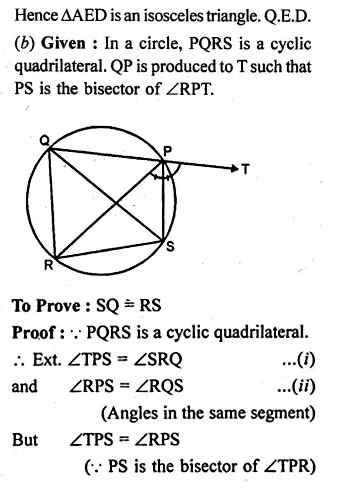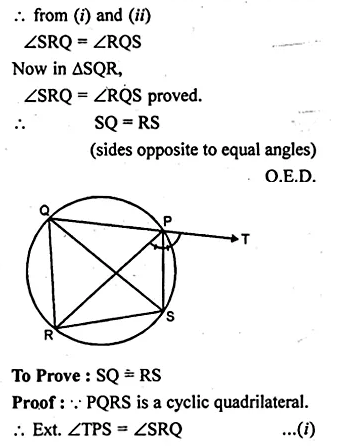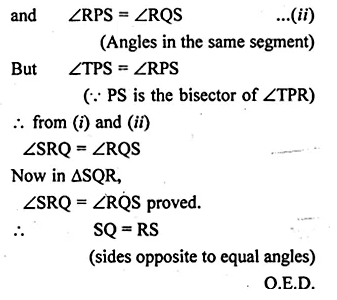Guru

# Ques 13(b) In the figure (ii) given below, SP is the bisector of ∠RPT and PQRS is a cyclic quadrilateral. Prove that SQ = RS.

• 0

This is circle based question from Chapter name- circles
Topic – Angle properties of circles
Chapter number- 15

In this question it is given that SP is the bisector of ∠RPT and PQRS is a cyclic quadrilateral.

And we have to Prove that SQ = RS.

ICSE Avichal publication
Understanding ICSE Mathematics
Question no 13(b)

Share

1.